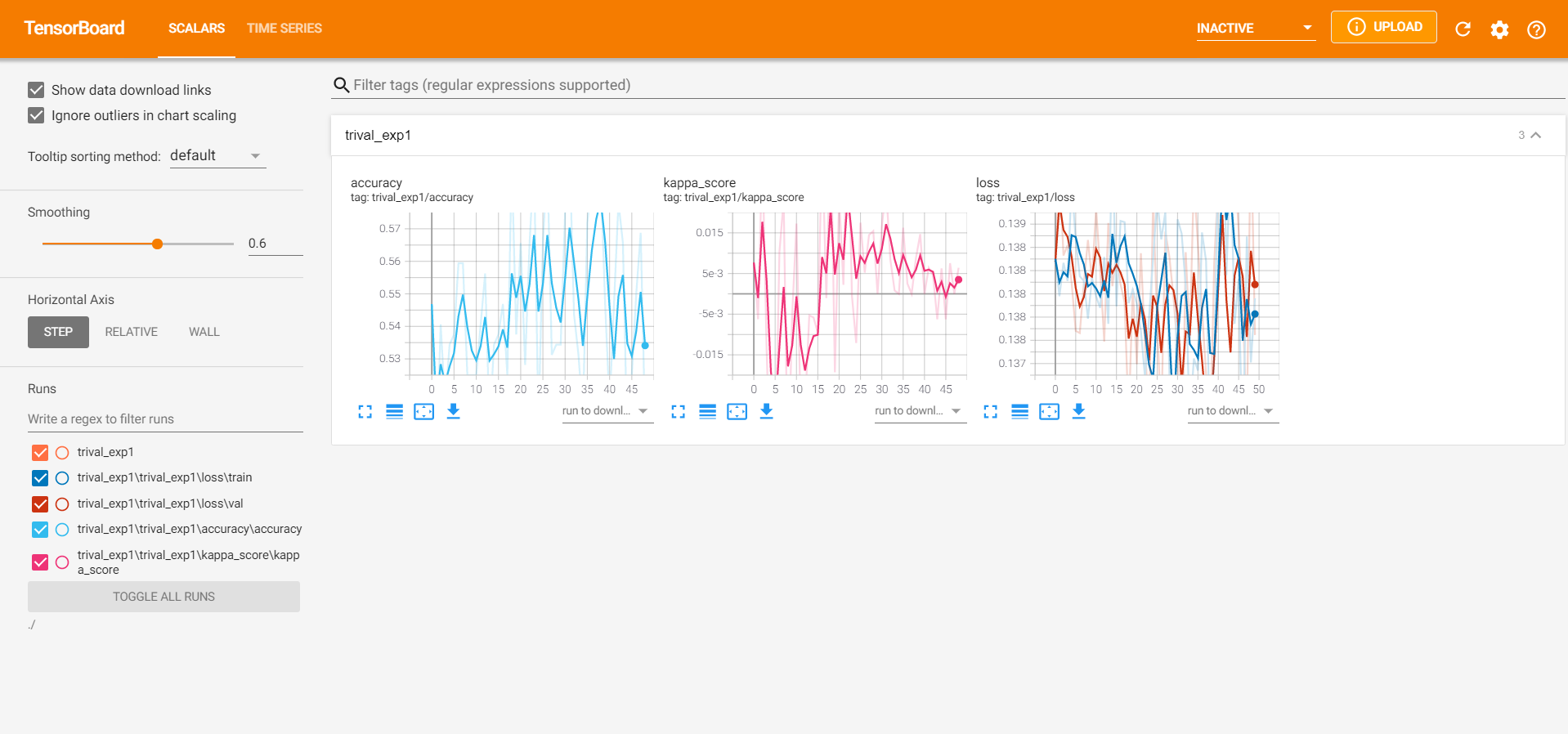# Minetorch教程

## 简介

“在Minecraft中，火把对于挖矿是非常重要的，没有火把玩家很难获得大量的钻石等矿物。在数据科学领域也是如此，一个名为PyTorch的深度学习工具库可以帮助我们获得数据中的钻石等宝藏。Minetorch则作为研究者的工具集，帮助我们以一个更加方便的方式使用PyTorch。”这是Minetorch的官方简介，说的直白一点，这是一个针对PyTorch的工具箱，包括了训练控制、训练日志记录及可视化等诸多功能。## 安装

pip install minetorch

## 使用教程

### 实验记录

trainer = Miner(
alchemistic_directory='./runs',  # 日志根目录
model=model,  # 模型
loss_func=loss_fn,  # 损失函数
optimizer=optimizer,  # 优化器
code="exp1",  # 实验子目录
resume=True,  # 是否自动加载之前的last模型接着训练
eval_stride=1,  # 多少轮进行一次评估
persist_stride=1,  # 多少轮进行一次checkpoint的保存
drawer='tensorboard',  # 绘图器设置
hooks=None,  # hooks配置
logging_format=None,  # 日志格式，默认即可
max_epochs=50,  # 训练轮数
plugins=[
MultiClassesClassificationMetricWithLogic(),
NoiseSampleDetector(metric=torch.nn.CrossEntropyLoss(reduction='none'))
],  # 插件
accumulated_iter=1,  # 累积多少次迭代后进行参数更新
trival=True,  # 是否同时完成训练后随机进行验证
in_notebook=True,  # 是否启用notebook模式，由于该库默认支持notebook，请务必打开该模式
amp=True,  # 是否开启混合精度
statable={}  # 实现了state_dict和load_state_dict方法的对象组成的字典，例如一个学习率调度器，trainer会自动保存这个对象的参数，注意优化器和模型默认是保存的因此不需要指定

)

### 训练控制

hook points 功能
after_init trainer初始化完成调用该方法
before_epoch_start 每轮开始时调用该方法
after_epoch_end 每轮结束时调用该方法
before_train_iteration_start 每次训练迭代开始之前调用该方法
after_train_iteration_end 每次训练迭代完成后调用该方法
before_val_iteration_start 每次验证迭代开始之前调用该方法
after_val_iteration_end 每次验证迭代完成之前调用该方法
before_checkpoint_persisted checkpoint保存前调用该方法
after_checkpoint_persisted checkpoint保存后调用该方法
before_quit 训练完成退出前调用该方法

def before_epoch_start(miner: Miner, **kwargs):
print("start training, this is epoch {}".format(miner.current_epoch))

trainer = Miner(..., hooks={'before_epoch_start': before_epoch_start})

hooks的设计使得训练的拓展变得上限极高，那么插件Plugin的存在则是更具体的一种实现，它的处理流程和hook函数类似，不同的是它封装了多个hook点的功能因而实现了一个更加完善的功能，例如上面代码示例中使用的MultiClassesClassificationMetricWithLogic即实现了多分类任务指标输出和drawer（通过miner获得drawer）绘制。设计新的插件需要注册自Plugin类然后实现自己不同hook点的方法。我这里就不具体讲解了，通过阅读作者实现的异常检测的plugin，应该可以理解设计的流程，我这里给出代码

### 训练演示

import torch
import torch.nn as nn
import torch.nn.functional as F
from minetorch.miner import Miner
from minetorch.metrics import MultiClassesClassificationMetricWithLogic
from minetorch.plugins.noise_detector import NoiseSampleDetector

# create dataset
x = torch.zeros(1600 * 10).view(1600, 10).float()
x = x + torch.rand_like(x)
y = torch.randint(low=0, high=2, size=(1600, ))

class MyDataset(Dataset):

def __init__(self, x, y):
assert x.shape == y.shape
self.x = x
self.y = y

def __getitem__(self, index):
return self.x[index], self.y[index]

def __len__(self):
return self.x.shape

# create model
class Model(nn.Module):
def __init__(self):
super(Model, self).__init__()
self.fc1 = nn.Linear(10, 100)
self.fc2 = nn.Linear(100, 2)

def forward(self, x):
x = self.fc1(x)
x = F.relu(x)
x = self.fc2(x)
return x

def before_epoch_start(miner: Miner, **kwargs):
print("start training, this is epoch {}".format(miner.current_epoch))

def main():
model = Model()
loss_fn = nn.CrossEntropyLoss()
optimizer = torch.optim.SGD(model.parameters(), lr=0.001)
# create trainer
trainer = Miner(
alchemistic_directory='./runs',
model=model,
loss_func=loss_fn,
optimizer=optimizer,
code="exp1",
resume=True,
eval_stride=1,
persist_stride=1,
drawer='tensorboard',
logging_format=None,
max_epochs=50,
plugins=[
MultiClassesClassificationMetricWithLogic(),
NoiseSampleDetector(metric=torch.nn.CrossEntropyLoss(reduction='none'))
],
accumulated_iter=1,
trival=True,
in_notebook=True,
amp=True,
hooks={'before_epoch_start': before_epoch_start},
)
trainer.train()

if __name__ == '__main__':
main()


└─trival_exp1
├─models
├─MultiClassesClassificationMetricWithLogic
├─NoiseSampleDetector
└─trival_exp1
├─accuracy
│  └─accuracy
├─kappa_score
│  └─kappa_score
└─loss
├─train
└─val目录